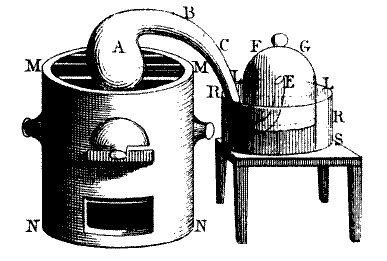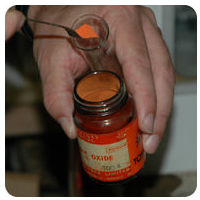# 11.5: Decomposition Reactions

$$\newcommand{\vecs}{\overset { \rightharpoonup} {\mathbf{#1}} }$$ $$\newcommand{\vecd}{\overset{-\!-\!\rightharpoonup}{\vphantom{a}\smash {#1}}}$$$$\newcommand{\id}{\mathrm{id}}$$ $$\newcommand{\Span}{\mathrm{span}}$$ $$\newcommand{\kernel}{\mathrm{null}\,}$$ $$\newcommand{\range}{\mathrm{range}\,}$$ $$\newcommand{\RealPart}{\mathrm{Re}}$$ $$\newcommand{\ImaginaryPart}{\mathrm{Im}}$$ $$\newcommand{\Argument}{\mathrm{Arg}}$$ $$\newcommand{\norm}{\| #1 \|}$$ $$\newcommand{\inner}{\langle #1, #2 \rangle}$$ $$\newcommand{\Span}{\mathrm{span}}$$ $$\newcommand{\id}{\mathrm{id}}$$ $$\newcommand{\Span}{\mathrm{span}}$$ $$\newcommand{\kernel}{\mathrm{null}\,}$$ $$\newcommand{\range}{\mathrm{range}\,}$$ $$\newcommand{\RealPart}{\mathrm{Re}}$$ $$\newcommand{\ImaginaryPart}{\mathrm{Im}}$$ $$\newcommand{\Argument}{\mathrm{Arg}}$$ $$\newcommand{\norm}{\| #1 \|}$$ $$\newcommand{\inner}{\langle #1, #2 \rangle}$$ $$\newcommand{\Span}{\mathrm{span}}$$$$\newcommand{\AA}{\unicode[.8,0]{x212B}}$$Figure $$\PageIndex{1}$$ (Credit: Madame Lavoisier, modified by User: Cdang/Wikimedia Commons; Source: http://commons.wikimedia.org/wiki/File:Lavoisier_decomposition_air.png(opens in new window); License: Public Domain)

### How does a decomposition reaction work?

Antoine Lavoisier is widely known as the “father of modern chemistry.” He was one of the first to study chemical reactions in detail. Lavoisier reacted mercury with oxygen to form mercuric oxide as part of his studies on the composition of the atmosphere. He was then able to show that the decomposition of mercuric oxide produced mercury and oxygen. The diagram above shows the apparatus used by Lavoisier to study the formation and decomposition of mercuric oxide.

## Decomposition Reactions

A decomposition reaction is a reaction in which a compound breaks down into two or more simpler substances. The general form of a decomposition reaction is:

$\ce{AB} \rightarrow \ce{A} + \ce{B}\nonumber$

Most decomposition reactions require an input of energy in the form of heat, light, or electricity.

Binary compounds are compounds composed of just two elements. The simplest kind of decomposition reaction is when a binary compound decomposes into its elements. Mercury (II) oxide, a red solid, decomposes when heated to produce mercury and oxygen gas.

$2 \ce{HgO} \left( s \right) \rightarrow 2 \ce{Hg} \left( l \right) + \ce{O_2} \left( g \right)\nonumber$Figure $$\PageIndex{2}$$: Mercury (II) oxide is a red solid. When it is heated, it decomposes into mercury metal and oxygen gas. (Credit: Ben Mills (User:Benjah-bmm27/Wikimedia Commons); Source: http://commons.wikimedia.org/wiki/File:Mercury%2528II%2529-oxide.jpg(opens in new window); License: Public Domain)

A reaction is also considered to be a decomposition reaction even when one or more of the products are still compounds. A metal carbonate decomposes into a metal oxide and carbon dioxide gas. For example, calcium carbonate decomposes into calcium oxide and carbon dioxide:

$\ce{CaCO_3} \left( s \right) \rightarrow \ce{CaO} \left( s \right) + \ce{CO_2} \left( g \right)\nonumber$

Metal hydroxides decompose on heating to yield metal oxides and water. Sodium hydroxide decomposes to produce sodium oxide and water:

$2 \ce{NaOH} \left( s \right) \rightarrow \ce{Na_2O} \left( s \right) + \ce{H_2O} \left( g \right)\nonumber$

Some unstable acids decompose to produce nonmetal oxides and water. Carbonic acid decomposes easily at room temperature into carbon dioxide and water:

$\ce{H_2CO_3} \left( aq \right) \rightarrow \ce{CO_2} \left( g \right) + \ce{H_2O} \left( l \right)\nonumber$

##### Exercise $$\PageIndex{1}$$

Can you build a balanced Chemical reaction for the decomposition of Potassium chlorate (KClO3)?

2KClO3 → 2KCl + 3O2

##### Example $$\PageIndex{1}$$: Decomposition Reactions

When an electric current is passed through pure water, it decomposes into its elements. Write a balanced equation for the decomposition of water.

###### Solution

Step 1: Plan the problem.

Water is a binary compound composed of hydrogen and oxygen. The hydrogen and oxygen gases produced in the reaction are both diatomic molecules.

###### Step 2: Solve.

The skeleton (unbalanced) equation:

$\ce{H_2O} \left( l \right) \overset{\text{elec}}{\rightarrow} \ce{H_2} \left( g \right) + \ce{O_2} \left( g \right)\nonumber$

Note the abbreviation "$$\text{elec}$$" above the arrow to indicate the passage of an electric current to initiate the reaction. Balance the equation.

$2 \ce{H_2O} \left( l \right) \overset{\text{elec}}{\rightarrow} 2 \ce{H_2} \left( g \right) + \ce{O_2} \left( g \right)\nonumber$

The products are elements and the equation is balanced.

##### Simulations

How does an airbag explode at just the right time in the event of a car accident? Learn more in this simulation:

How does a chemical reaction inflate and airbag

## Summary

• A definition of decomposition reaction and example reactions are given.

## Review

1. What is a decomposition reaction?
2. What is usually needed for a decomposition reaction to take place?
3. Are elements always the product of a decomposition reaction?

This page titled 11.5: Decomposition Reactions is shared under a CK-12 license and was authored, remixed, and/or curated by CK-12 Foundation via source content that was edited to the style and standards of the LibreTexts platform; a detailed edit history is available upon request.